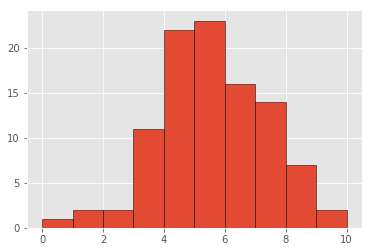## Basic Statistics in Python

• Post author:
• Post category:Python

# Basic Statistics in Python

Let’s create a dataset to work with and plot a histogram to visualise:

In :
import numpy as np
from scipy import stats

import matplotlib
import matplotlib.pyplot as plt
%matplotlib inline
matplotlib.style.use('ggplot')

np.random.seed(1)
data = np.round(np.random.normal(5, 2, 100))
plt.hist(data, bins=10, range=(0,10), edgecolor='black')
plt.show()# Measures of Central Tendency

Our measures of central tendency include mean, median or mode

The mean is calculated as

$$\mu = \dfrac{\sum_{i=1}^{N} x_i}{N}$$

The median value is the middlemost value, you take the value in the position of

$$\dfrac{n+1}{2}$$

of the sorted data.

The mode is the most frequent value.

### Mean

Numpy implements a mean function for calculating the mean:

In :
mean = np.mean(data)
mean

Out:
5.0999999999999996

### Median

Numpy also implements a median function for calculating the median:

In :
np.median(data)

Out:
5.0

### Mode

We can see from our histogram already that 5 is the modal value.

There is no in-built numpy mode function, but there is one from the scipy stats module we can use.

In :
mode = stats.mode(data)

print("The modal value is {} with a count of {}".format(mode.mode, mode.count))

The modal value is 5.0 with a count of 23


# Range

The range gives a measure of how spread apart the values are.

The range is simply calculated as the maximum value – minimum value

$$Max(x_i) – Min(x_i)$$

Numpy implements this as a point to point function np.ptp.

In :
np.ptp(data)

Out:
9.0

# Variance

Variance is a measure of how variable the data is, it is calculated as:

$$\sigma^2 = \dfrac{\sum_{i=1}^{N} (x_i – \mu)^2}{N}$$

Numpy implements the variance as a function np.var()

In :
np.var(data)

Out:
3.0699999999999998

# Standard Deviation

The variance can get very large for large data sets and so we will often use the standard deviation, which is the square root of the variance:

$$\sigma = \sqrt{\sigma^2}$$

68.2% of the data falls within 1 standard deviation of the mean, 95.4% falls within 2 standard deviations of the mean, and 99.7% falls within 3 standard deviations.

This is implemented in Numpy as np.std()

In :
np.std(data)

Out:
1.7521415467935231

# Standard Error

The standard error of the mean (SE of the mean) estimates the variability between sample means that you would obtain if you took multiple samples from the same population. The standard error of the mean estimates the variability between samples whereas the standard deviation measures the variability within a single sample.

It is calculated as:

$$SE = \dfrac{s}{\sqrt{n}}$$

Where s is the sample standard deviation. Again Numpy doesn’t have an implementation of this (though it is easy to calculate), but we can use scipy’s stats module instead:

In :
stats.sem(data)

Out:
0.1760968512214259

So had we taken multiple samples from the same population we would expect the standard deviation of the means of those samples to be 0.176.